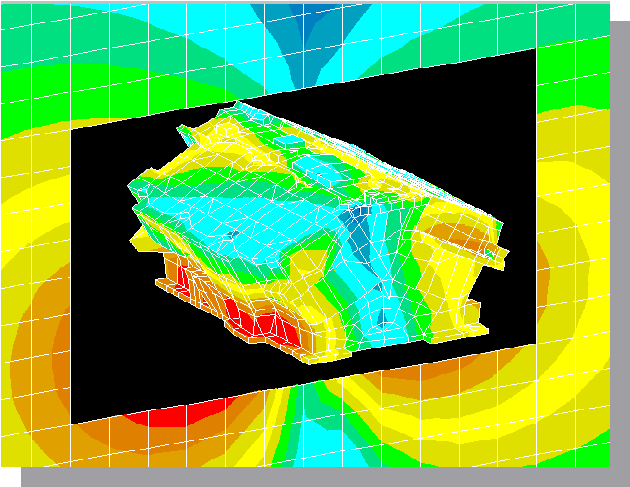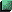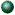# Boundary Element Method

## Course Numbers: 20-MECH-7504;   Spring Semester

Instructor: Dr. Yijun Liu, Professor of Mechanical EngineeringIntroducing the CourseCourse AnnouncementCourse Syllabus and ReferencesBEM Syllabus; Reserved Reference BooksContents of the LecturesChapter 1. Introduction

• Lecture 1. Introduction to the BEM
• Lecture 2. An Example - Bending of a Simple Beam
• Lecture 3. Review of the Mathematical Principles
• Homework #1Chapter 2. Potential Problem

• Lecture 1. Governing Equation and the Physical Problems; the Fundamental Solutions (2-D and 3-D)
• Lecture 2. Boundary Integral Equation (BIE) Formulation
• Lecture 3. Discretization of the BIE Using Constant Boundary Elements
• Lecture 4. Higher Order Boundary Elements
• Lecture 5. Multi-Region Problems; Infinite Domain Problems
• Lecture 6. Domain Integrals; Indirect BIE/BEM Formulations
• Lecture 7. Programming for the BEM
• Lecture 8. Applications of the BEM for Potential Problems
• Homework #2; Homework #3
• BEM Program for 2-D Potential Problems (include the PC executable file; Fortran source code and an input file)Chapter 3. Fast Multipole BEM for Potential Problem

• Lecture 1. Introduction FMM
• Lecture 2. FMM for 2-D Potential Problems
• Lecture 3. Programming of Fast Multipole BEM for 2-D Potential Problem
• Lecture 4. Applications
• Midterm Project AssignmentChapter 4. Elastostatic Problem

• Lecture 1. Governing Equations and the Fundamental Solutions
• Lecture 2. BIE Formulation
• Lecture 3. Fast multipole BEM for Elastostatic Problem
• Lecture 4. Applications in Stress Analysis and Fracture Mechanics Problems
• Homework #4Chapter 5. Stokes Flow Problem

• Lecture 1. Governing Equations and the Fundamental Solutions
• Lecture 2. BIE Formulation
• Lecture 3. Fast multipole BEM for Stokes Flow Problem
• Lecture 4. Applications
• Homework #5Chapter 6. Acoustic Problem

• Lecture 1. Basic Equations in Acoustics and the BIE Formulation
• Lecture 2. Advanced BIE Formulations in Acoustics
• Lecture 3. Fast multipole BEM for Acoustic Problem
• Lecture 4. Applications in Structural Acoustics
• Homework #6
• Final ProjectChapter 7. Further Research Topics

• Lecture 1. Recent development in the BEM
• Presentation of the Final Project - I
• Presentation of the Final Project - II

Contact Info

E-mail:   Yijun.Liu@uc.edu
Tel.
:         (513) 556-4607 (Voice),    (513) 556-3390 (Fax)
S-mail:    Mechanical Engineering, University of Cincinnati, P.O. Box 210072, Cincinnati, OH 45221-0072

 ©  1997-2013 Yijun Liu, University of Cincinnati Last updated April 26, 2013.